Python Exemplary
deutsch     english

## STEPPER MOTORS### How stepper motors work

The rotor of a stepper motor is like a compass needle, one side is the north, the other side is the south pole. The north pole of a magnetic needle turns in the direction of a magnetic field H like the compass needle points to the north in the earth magnetic field.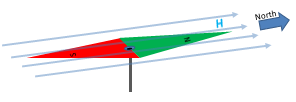In a stepper motor a magnetic field is created by two coils A and B in a perpendicular magnet arrangement. The rotor is in the gap of the two magnets and acts like a magnetic needle oriented in the direction of the resulting magnetic field that is a superposition (vector addition) of the two fields.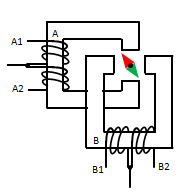The two coils A and B have both a center tap connected to VCC. Since the direction of the magnetic field is a right-hand thread of the current in the windings, the direction of the magnetic field can be switched by pulling to ground (GND) one or the other side of each coil. (Current flows from VCC to GND, see red arrows).

To turn the rotor, the resulting field is rotated in 90 degrees steps by selecting the right sequence of coil currents. As you can see in the diagrams below, to create a counter-clockwise rotation, the 4 inputs A1, A2, B2, B2 must be connected as follows:

 A1 A2 B1 B2 Direction (deg) GND open GND open 45 open GND GND open 135 open GND open GND 225 GND open open GND 315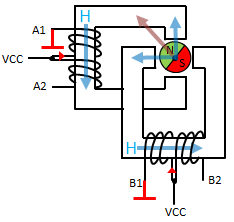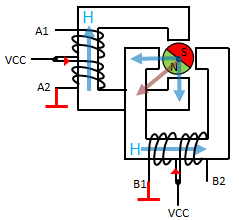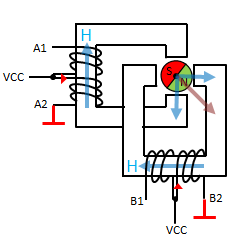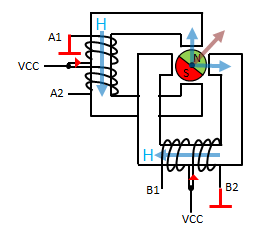Blue arrows: Magnetic field of each coil (alongside the magnet arms).
The resulting magnetic field in the gap is the superposition of the two fields (brown arrow).

### Experiment 1: Stepper motor connected via a ULN2003A driver

Aim:
Connect a small stepper motor to the Raspberry Pi and and turn the shaft continuously 180 degrees forward and backward.

Circuitry:
Never connect a stepper motor directly to the GPIO, but use a current driver made by MOSFETs, bipolar transistors or the L293D. For small motors the Darlington Array ULN2003A driver is also a good choice. Because it is an inverting (open collector) amplifier, a high input signal pulls the driver output to GND, while a low input sets the output to high impedance (like open).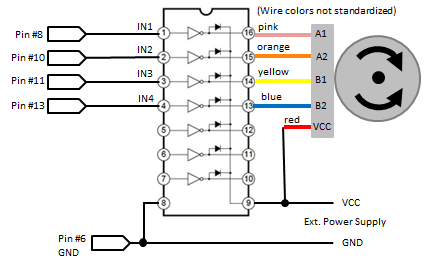If you compare the circuit to the table above, you find the following correspondence:

 IN1 IN2 IN3 IN4 Direction (deg) 1 0 1 0 45 0 1 1 0 135 0 1 0 1 225 1 0 0 1 315

0 = LOW, 1 = HIGH

If setStepper(in1, in2, in3, in4) is a function that applies the logic values to the driver inputs, a full counter-clockwise rotation of the rotor can be obtained by calling the sequence:

def forwardStep():
setStepper(1, 0, 1, 0)
setStepper(0, 1, 1, 0)
setStepper(0, 1, 0, 1)
setStepper(1, 0, 0, 1)

Normally there is a gear that links the rotor axis and the motor shaft. So the number of forwardStep() to turn the shaft for a given angle depends on the reduction ratio of the gear. In this example, 512 calls are needed for a 360 degrees shaft rotation.

Program:[►]

```# Stepper1.py

import RPi.GPIO as GPIO
import time

P_A2 = 10 # ditto
P_B1 = 11 # ditto
P_B2 = 13 # ditto
delay = 0.005 # time to settle

def setup():
GPIO.setmode(GPIO.BOARD)
GPIO.setup(P_A1, GPIO.OUT)
GPIO.setup(P_A2, GPIO.OUT)
GPIO.setup(P_B1, GPIO.OUT)
GPIO.setup(P_B2, GPIO.OUT)

def forwardStep():
setStepper(1, 0, 1, 0)
setStepper(0, 1, 1, 0)
setStepper(0, 1, 0, 1)
setStepper(1, 0, 0, 1)

def backwardStep():
setStepper(1, 0, 0, 1)
setStepper(0, 1, 0, 1)
setStepper(0, 1, 1, 0)
setStepper(1, 0, 1, 0)

def setStepper(in1, in2, in3, in4):
GPIO.output(P_A1, in1)
GPIO.output(P_A2, in2)
GPIO.output(P_B1, in3)
GPIO.output(P_B2, in4)
time.sleep(delay)

setup()
while True:
print "forward"
for i in range(256):
forwardStep()
print "backward"
for i in range(256):
backwardStep()
```
Highlight program code (Ctrl+C copy, Ctrl+V paste)

Remarks:
If the settle time delay is too short, the motor has not enough time to reach the new position and the result is disastrous.